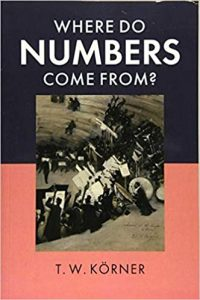Les comptes-rendus de livres présentent au lectorat de la SMC des ouvrages intéressants sur les mathématiques et l’enseignement des mathématiques dans un large éventail de domaines et sous-domaines.Vos commentaires, suggestions et propositions sont les bienvenues.

Karl Dilcher, Université Dalhousie (notes-reviews@cms.math.ca)Where Do Numbers Come From?
by T. W. Körner
Cambridge University Press, 2019
ISBN: 978-1-108-73838-5
Reviewed by Karl Dilcher

A concise and accurate description of the purpose and contents of this book is given by the text on the back cover of this paperback, which I’ll quote in full:

Why do we need the real numbers? How should we construct them? These questions arose in the nineteenth century, along with the ideas and techniques needed to address them. Nowadays it is commonplace for apprentice mathematicians to hear ‘we shall assume the standard properties of the real numbers’ as part of their training. But exactly what are those properties? And why can we assume them?

This book is clearly and entertainingly written for those students, with historical asides and exercises to foster understanding. Starting with the natural (counting) numbers and then looking at the rational numbers (fractions) and negative numbers, the author builds to a careful construction of the real numbers followed by the complex numbers, leaving the reader fully equipped with all the number systems required by modern mathematical analysis. Additional chapters on polynomials and quarternions provide further context for any reader wanting to delve deeper.

The author acknowledges a few older essays and books that cover some of the same ground. I quote from the Introduction:

« The question ‘What are numbers?’ […] has interested several important philosophers and mathematicians. The answer given in this books is essentially that given by Dedekind in two essays, ‘Stetigkeit und irrationale Zahlen’ (‘Continuity and irrational numbers’) and ‘Was sind und was sollen die Zahlen’ (‘What are numbers and what should they be?’) » Later in the Introduction the author writes, « If the reader finds this text too verbose or insufficiently precise, she should read Landau’s Foundations of Analysis, a little gem of a book which covers the same ground with great precision and without a wasted word. »

Some of the topics that go beyond strictly developing the number systems are worth mentioning: The section ‘Some Pretty Theorems’ deals with Fermat’s little theorem and Wilson’s theorem, after a brief introduction to finite fields. The section ‘A New Use for Old Numbers’ begins with the Morse code and proceeds to Hamming codes. Other sections with intriguing titles include ‘Mathematics Becomes a Profession’, ‘How Can We Justify Calculus?’, and ‘Are the Real Numbers Real?’

The book contains numerous exercises, and I like the author’s advice to “read the statements of the exercises carefully, but work through only the ones that interest [you]”. Sketch solutions to most exercises can be found on the author’s homepage.

« Where do numbers come from? » is a question every mathematician should ask, and hopefully every student as well. The answer to this, and many other interesting questions and problems, can be found in this engaging book.